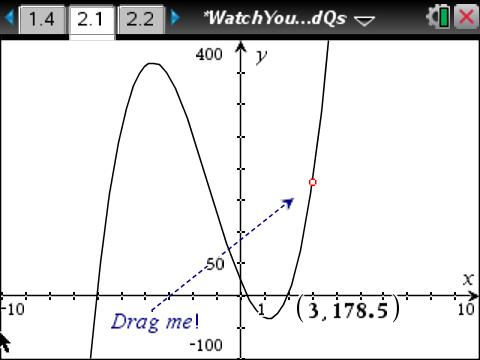••• ##### Device
•TI-Nspire™ CX/CX II
•TI-Nspire™ CX CAS/CX II CAS
• TI-Nspire™ Navigator™
• ##### Software

TI-Nspire™ CX
TI-Nspire™ CX CAS

5.0

• ##### Report an Issue

Algebra 2: Watch Your P' and Q's
by Texas Instruments

#### Overview

Students use the Rational Zero Theorem to find all rational zeros of a polynomial.

#### Key Steps

•Students will investigate the Rational Zero Theorem. Students will identify the number of real roots by graphing, and use the graph to approximate the real roots.

•Students will then use the Rational Zero Theorem to find all the potential rational zeros of the function. Using the calculator, they will use a list to calculate the y value for each of the potential zeros to find the actual rational zeros.

•Encourage students to summarize what they have learned from completing the activity.